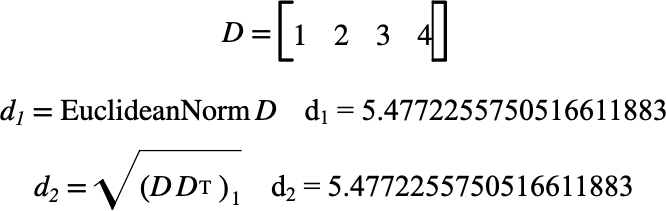# $$\text{EuclideanNorm}$$¶

You can use the $$\text{EuclideanNorm}$$ to calculate the Euclidean norm of a matrix defined by:

$\left \Vert X \right \Vert _ 2 = \sqrt{ \sum_{r=1}^{nrows \left ( X \right ) } \sum_{c=1}^{ncols \left ( X \right ) } \left ( X _ { r,c } \right ) ^ 2 }$

You can use the \enorm backslash command to insert this function.

The following variants of this function are available:

• $$\text{real } \text{EuclideanNorm} \left ( \text{<matrix>} \right )$$

Where $$\text{<matrix>}$$ can be any matrix type.

The function is implemented internally for real and complex matrices. Other matrix types will be converted to real matrices prior to calculating the norm.

Figure 127 shows the basic use of the $$\text{EuclideanNorm}$$ function.Figure 127 Example Use Of The EuclideanNorm Function